# RD Sharma Solutions for Class 10 Maths Chapter 8 Quadratic Equations Exercise 8.7

Quadratic Equations are found in many areas of study and applications. This exercise briefs students on problems involving quadratic equations. Students can clear any conceptual doubts by referring to RD Sharma Solutions Class 10. Additionally, students can also utilise the RD Sharma Solutions for Class 10 Maths Chapter 8 Quadratic Equations Exercise 8.7 PDF given below.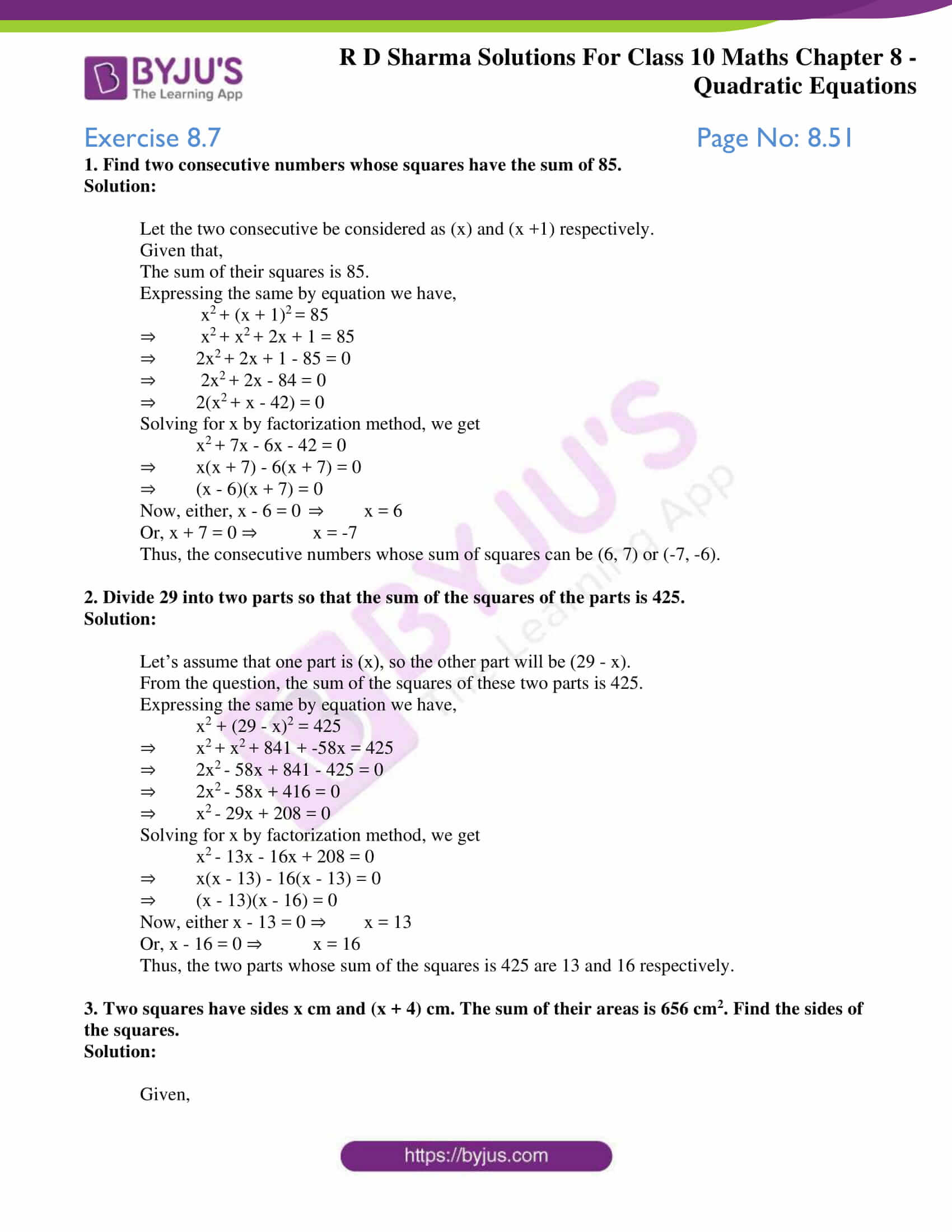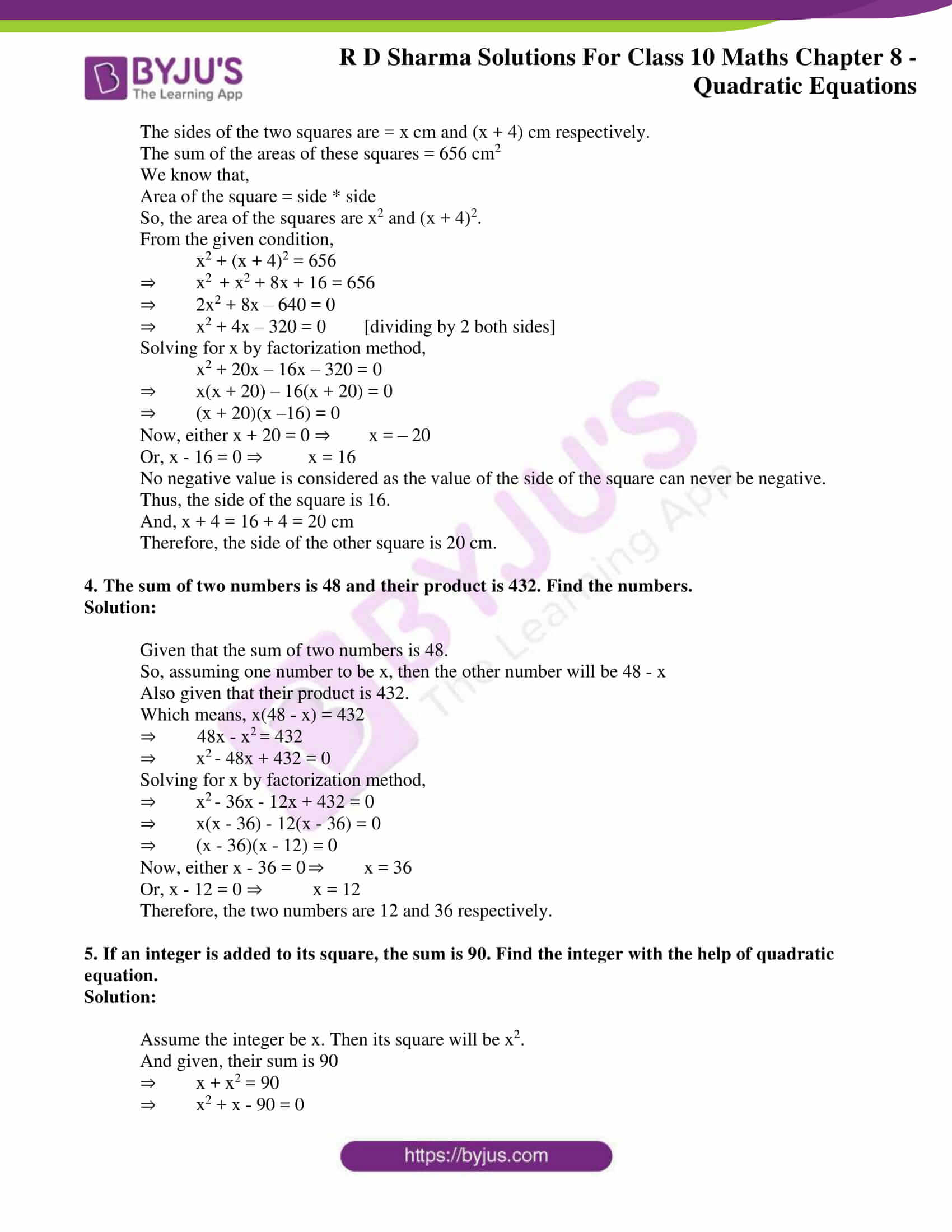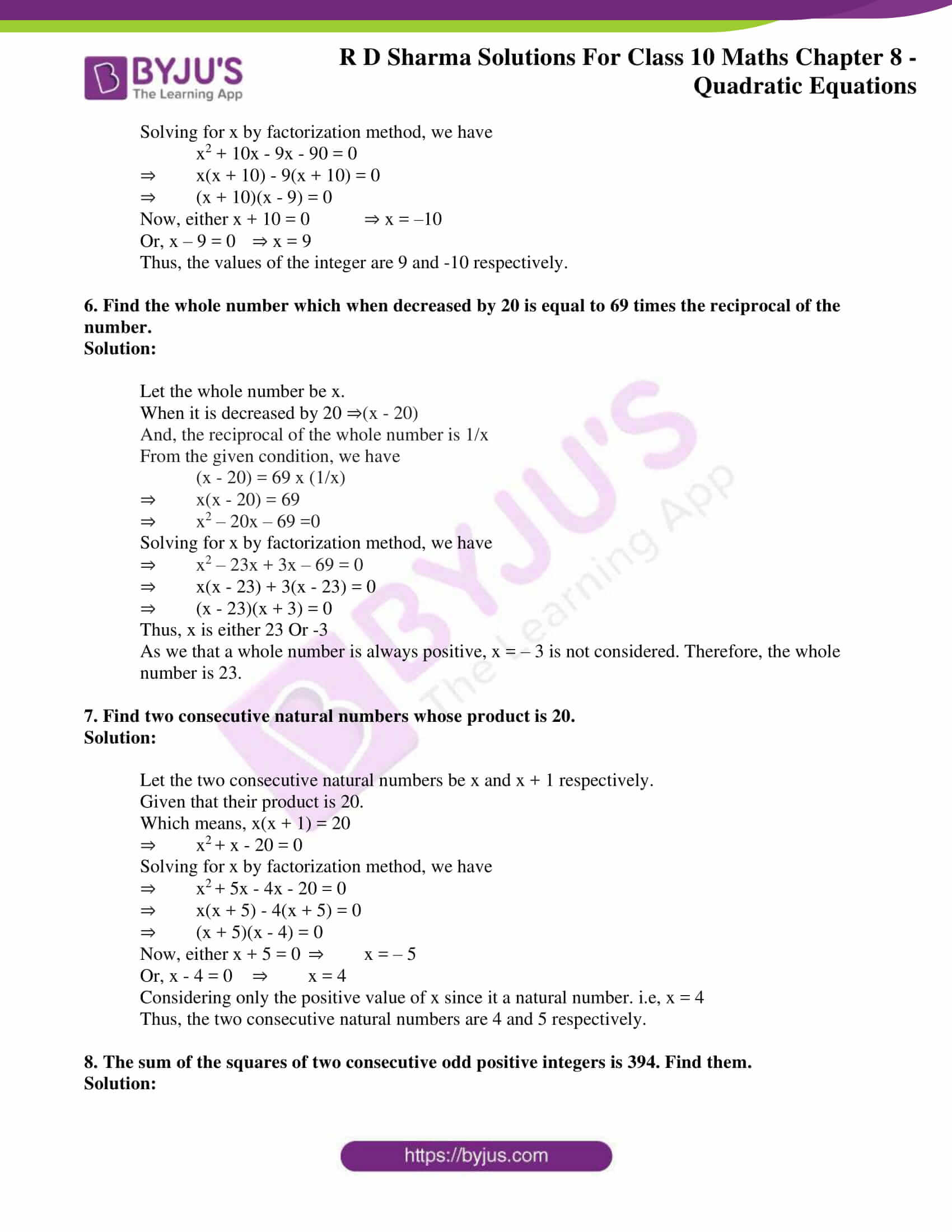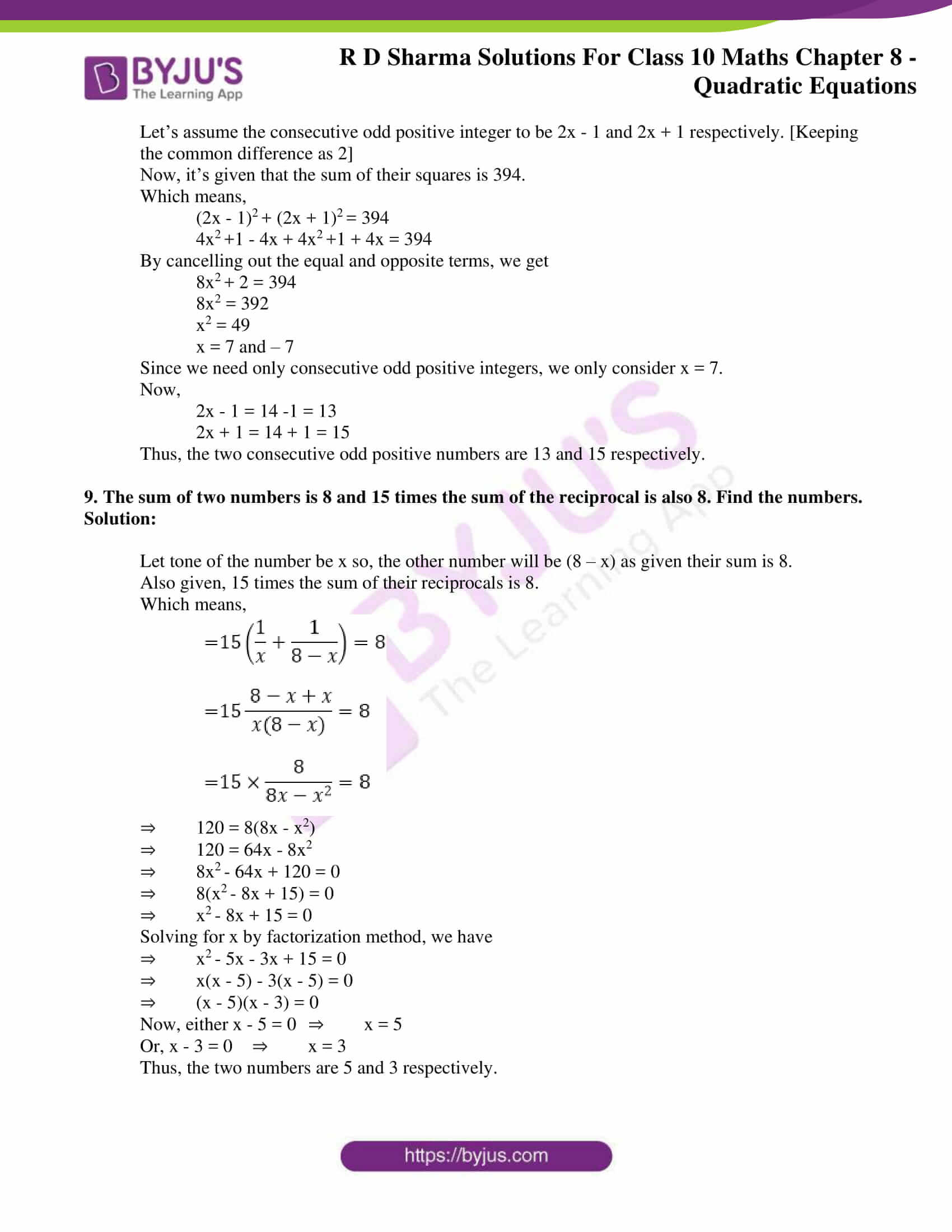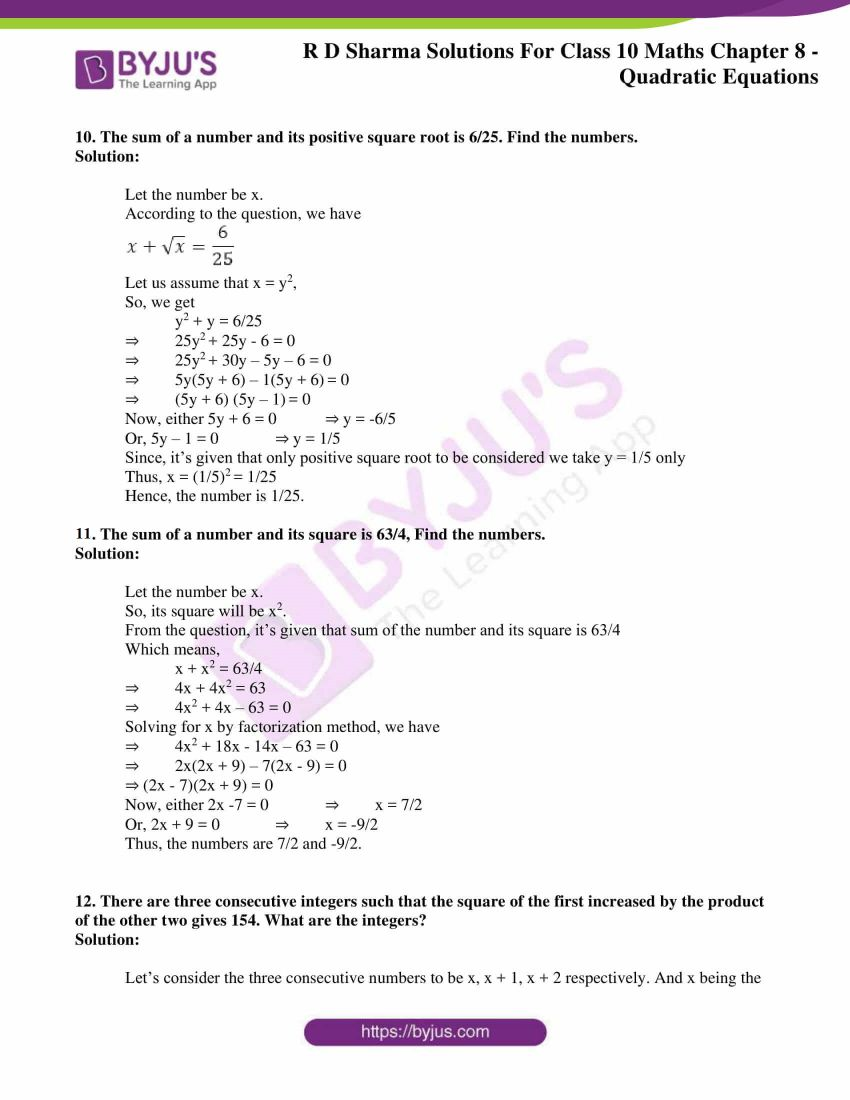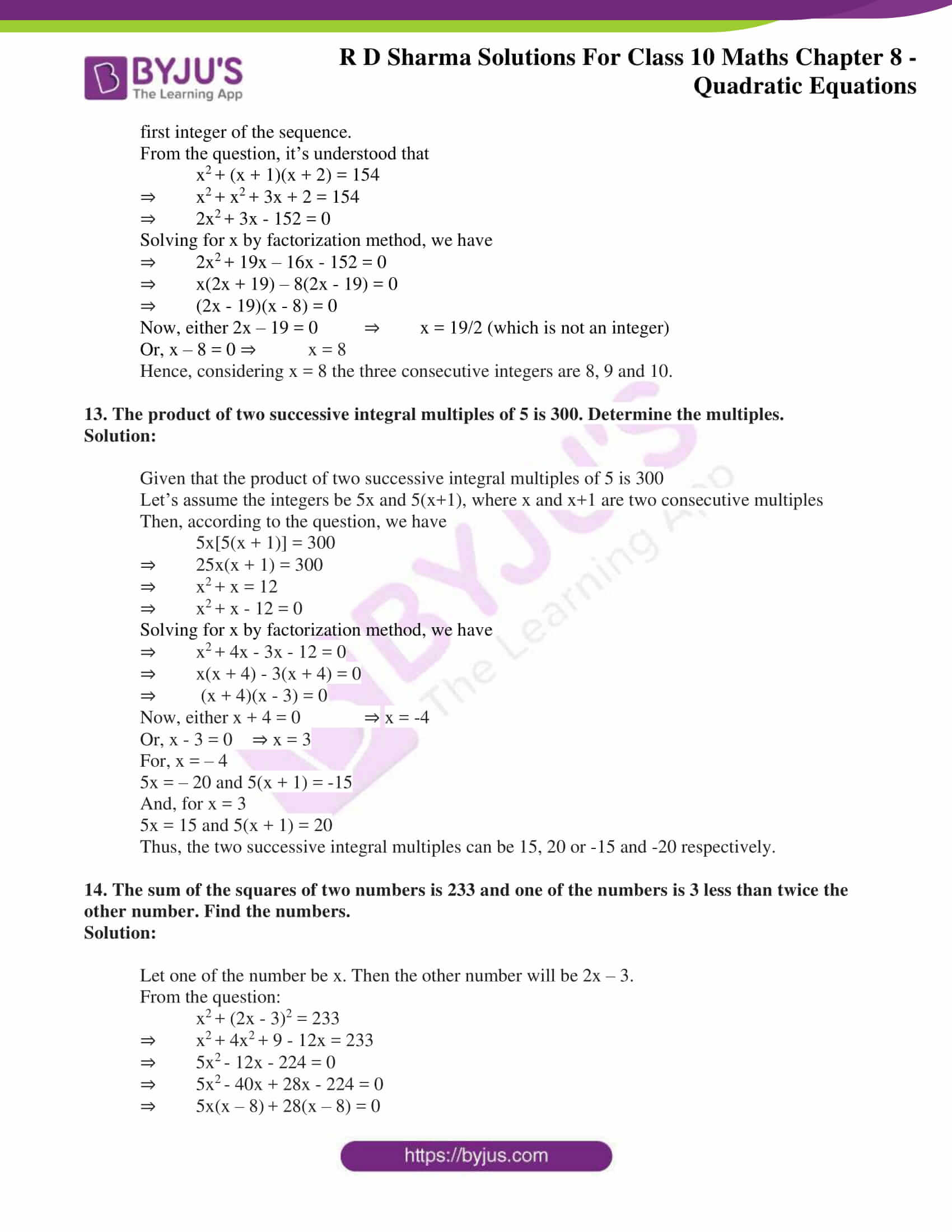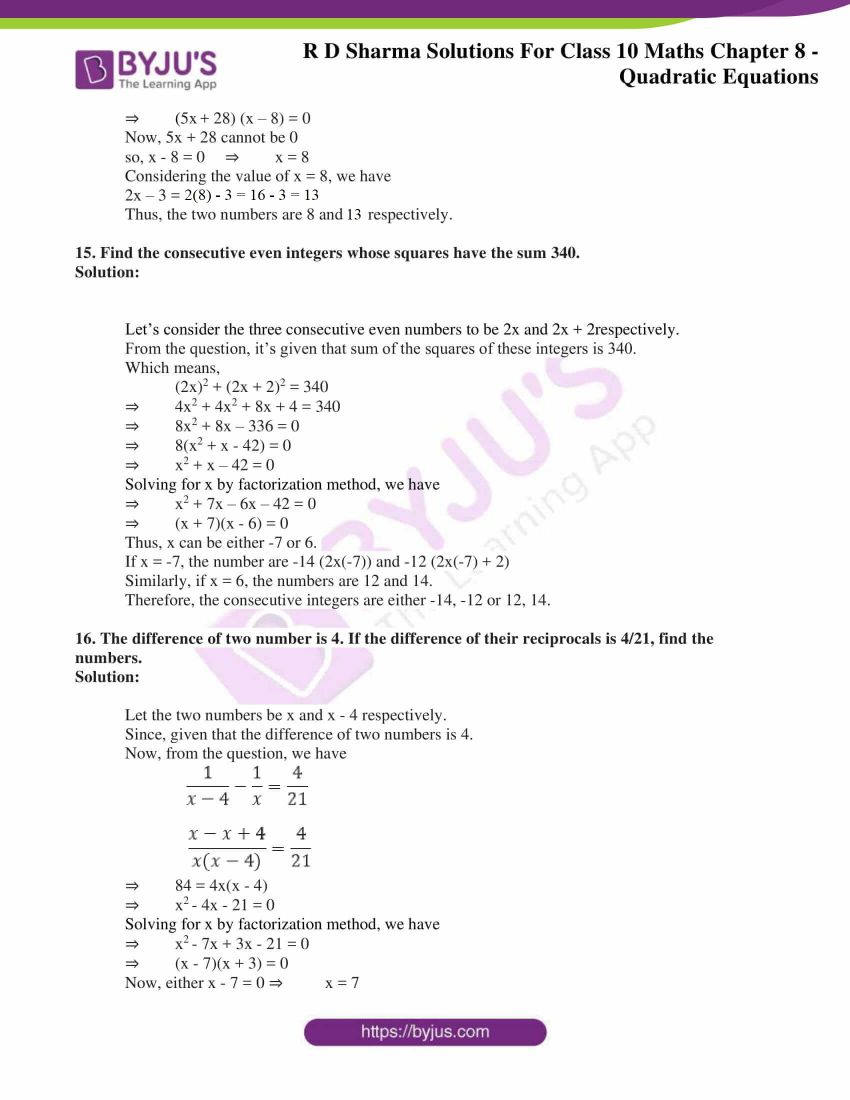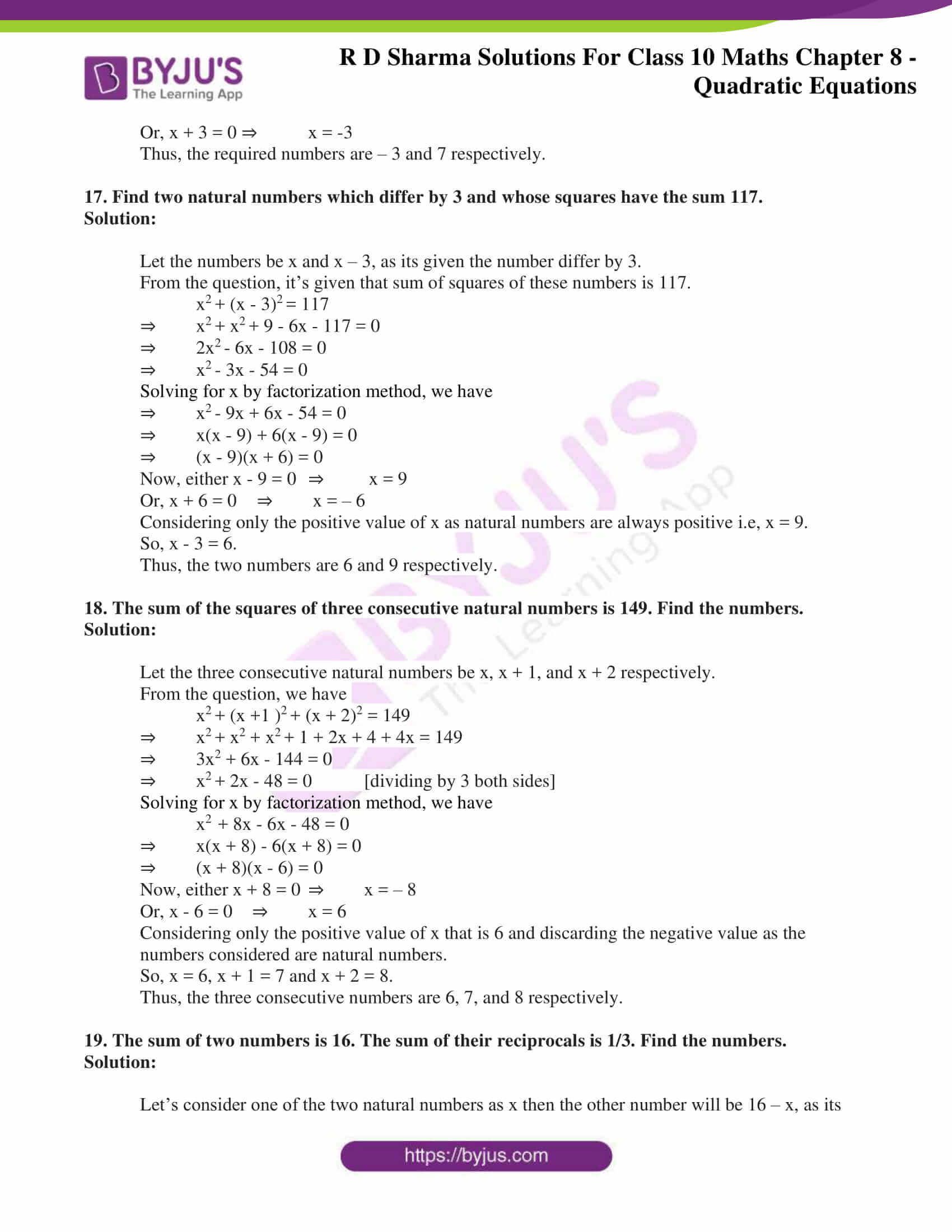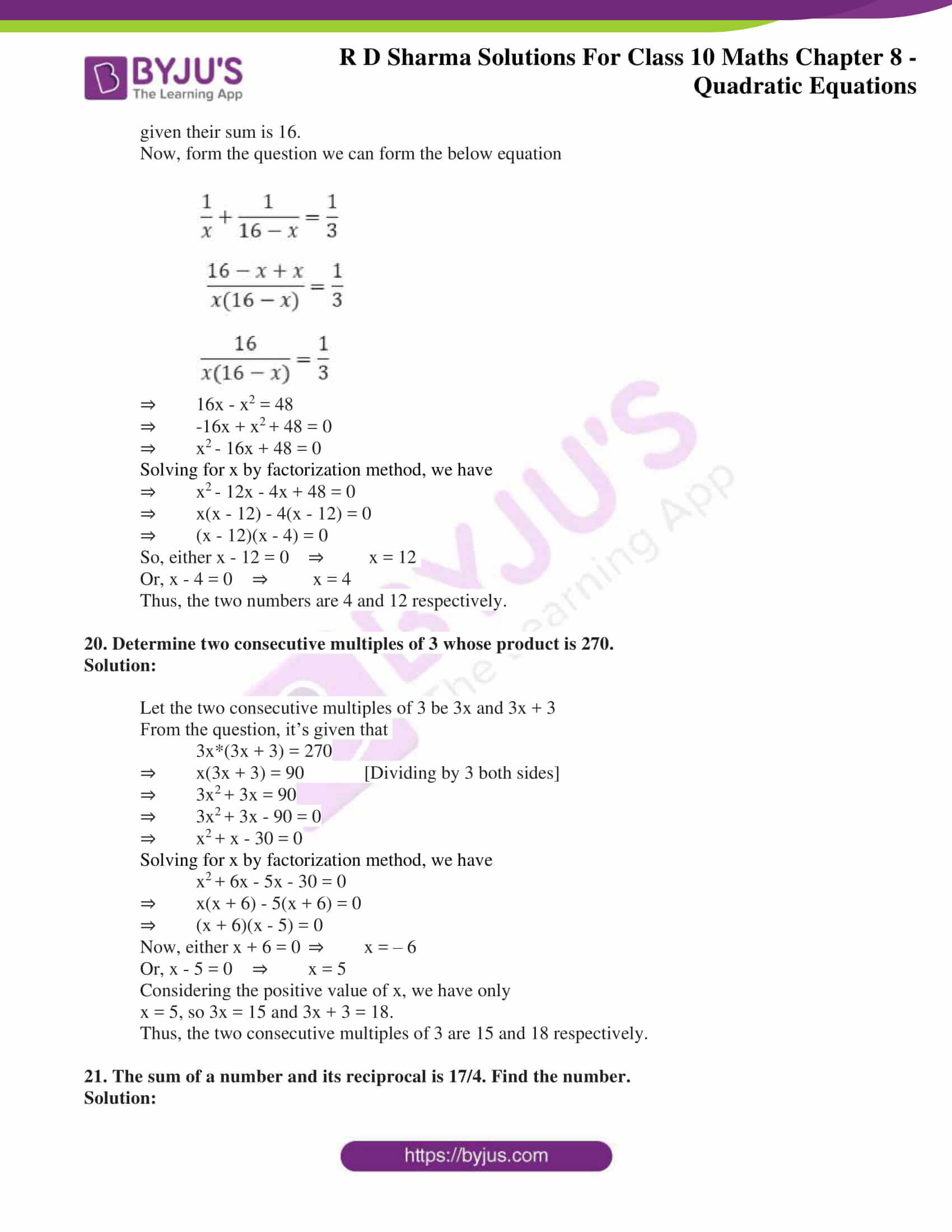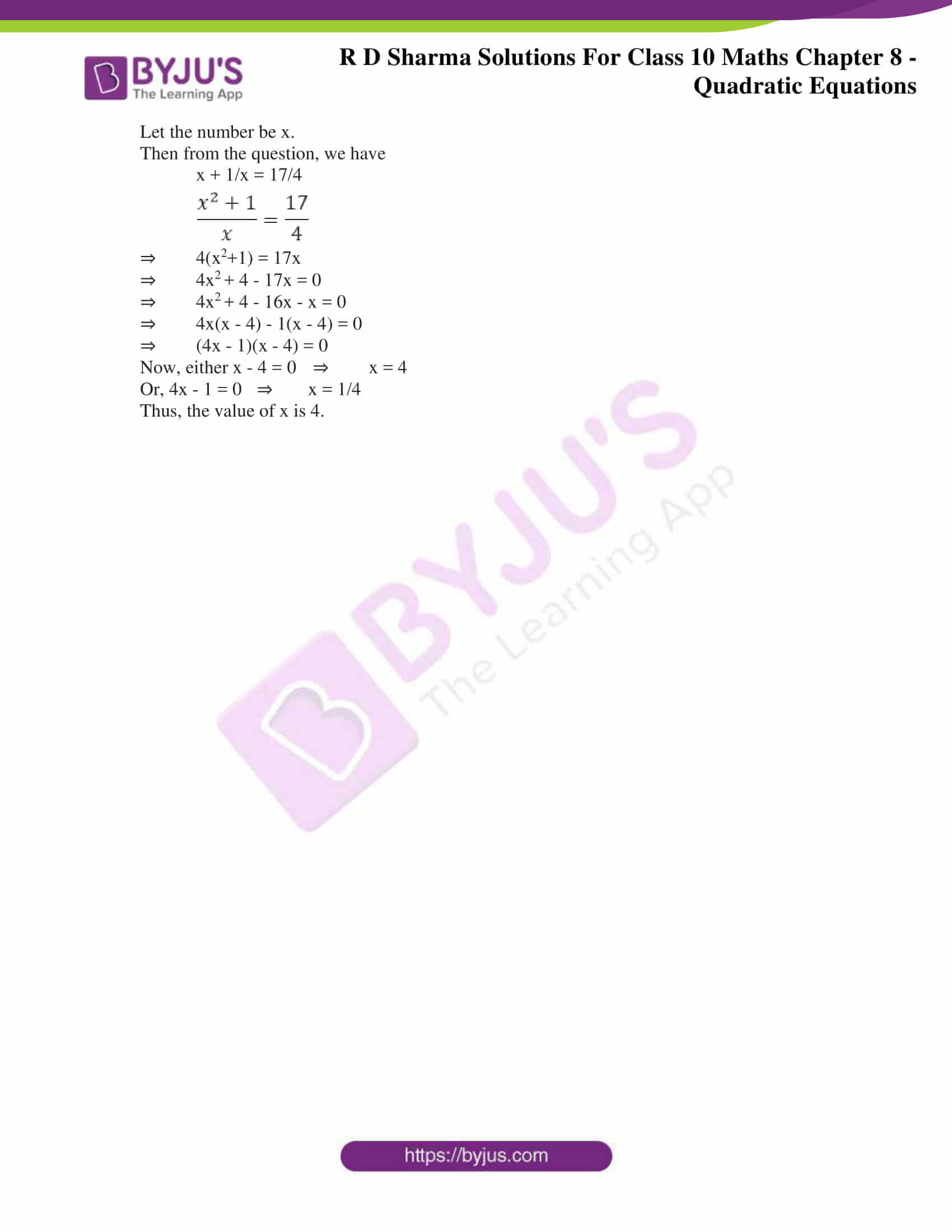### Access RD Sharma Solutions for Class 10 Maths Chapter 8 Quadratic Equations Exercise 8.7

1. Find two consecutive numbers whose squares have the sum of 85.

Solution:

Let the two consecutive be considered as (x) and (x +1) respectively.

Given that,

The sum of their squares is 85.

Expressing the same by equation we have,

x+ (x + 1)= 85

⇒ x+ x+ 2x + 1 = 85

⇒ 2x+ 2x + 1 – 85 = 0

⇒ 2x+ 2x – 84 = 0

⇒ 2(x+ x – 42) = 0

Solving for x by factorization method, we get

x+ 7x – 6x – 42 = 0

⇒ x(x + 7) – 6(x + 7) = 0

⇒ (x – 6)(x + 7) = 0

Now, either, x – 6 = 0  ⇒ x = 6

Or, x + 7 = 0 ⇒ x = -7

Thus, the consecutive numbers whose sum of squares can be (6, 7) or (-7, -6).

2. Divide 29 into two parts so that the sum of the squares of the parts is 425.

Solution:

Let’s assume that one part is (x), so the other part will be (29 – x).

From the question, the sum of the squares of these two parts is 425.

Expressing the same by equation we have,

x2 + (29 – x)2 = 425

⇒ x+ x+ 841 + -58x = 425

⇒ 2x– 58x + 841 – 425 = 0

⇒ 2x– 58x + 416 = 0

⇒ x– 29x + 208 = 0

Solving for x by factorization method, we get

x– 13x – 16x + 208 = 0

⇒ x(x – 13) – 16(x – 13) = 0

⇒ (x – 13)(x – 16) = 0

Now, either x – 13 = 0 ⇒ x = 13

Or, x – 16 = 0 ⇒ x = 16

Thus, the two parts whose sum of the squares is 425 are 13 and 16 respectively.

3. Two squares have sides x cm and (x + 4) cm. The sum of their areas is 656 cm2. Find the sides of the squares.

Solution:

Given,

The sides of the two squares are = x cm and (x + 4) cm respectively.

The sum of the areas of these squares = 656 cm2

We know that,

Area of the square = side * side

So, the area of the squares are x2 and (x + 4)2.

From the given condition,

x2 + (x + 4)2 = 656

⇒ x2 + x2 + 8x + 16 = 656

⇒ 2x2 + 8x – 640 = 0

⇒ x2 + 4x – 320 = 0 [dividing by 2 both sides]

Solving for x by factorization method,

x2 + 20x – 16x – 320 = 0

⇒ x(x + 20) – 16(x + 20) = 0

⇒ (x + 20)(x –16) = 0

Now, either x + 20 = 0 ⇒ x = – 20

Or, x – 16 = 0 ⇒ x = 16

No negative value is considered as the value of the side of the square can never be negative.

Thus, the side of the square is 16.

And, x + 4 = 16 + 4 = 20 cm

Therefore, the side of the other square is 20 cm.

4. The sum of two numbers is 48 and their product is 432. Find the numbers.

Solution:

Given that the sum of two numbers is 48.

So, assuming one number to be x, then the other number will be 48 – x

Also given that their product is 432.

Which means, x(48 – x) = 432

⇒ 48x – x= 432

⇒ x– 48x + 432 = 0

Solving for x by factorization method,

⇒ x– 36x – 12x + 432 = 0

⇒ x(x – 36) – 12(x – 36) = 0

⇒ (x – 36)(x – 12) = 0

Now, either x – 36 = 0 ⇒ x = 36

Or, x – 12 = 0 ⇒ x = 12

Therefore, the two numbers are 12 and 36 respectively.

5. If an integer is added to its square, the sum is 90. Find the integer with the help of quadratic equation.

Solution:

Assume the integer be x. Then its square will be x2.

And given, their sum is 90

⇒ x + x2 = 90

⇒ x2 + x – 90 = 0

Solving for x by factorization method, we have

x2 + 10x – 9x – 90 = 0

⇒ x(x + 10) – 9(x + 10) = 0

⇒ (x + 10)(x – 9) = 0

Now, either x + 10 = 0 ⇒ x = –10

Or, x – 9 = 0 ⇒ x = 9

Thus, the values of the integer are 9 and -10 respectively.

6. Find the whole number which when decreased by 20 is equal to 69 times the reciprocal of the number.

Solution:

Let the whole number be x.

When it is decreased by 20 ⇒(x – 20)

And, the reciprocal of the whole number is 1/x

From the given condition, we have

(x – 20) = 69 x (1/x)

⇒ x(x – 20) = 69

⇒ x2 – 20x – 69 =0

Solving for x by factorization method, we have

⇒ x2 – 23x + 3x – 69 = 0

⇒ x(x – 23) + 3(x – 23) = 0

⇒ (x – 23)(x + 3) = 0

Thus, x is either 23 Or -3

As we that a whole number is always positive, x = – 3 is not considered. Therefore, the whole number is 23.

7. Find two consecutive natural numbers whose product is 20.

Solution:

Let the two consecutive natural numbers be x and x + 1 respectively.

Given that their product is 20.

Which means, x(x + 1) = 20

⇒ x+ x – 20 = 0

Solving for x by factorization method, we have

⇒ x+ 5x – 4x – 20 = 0

⇒ x(x + 5) – 4(x + 5) = 0

⇒ (x + 5)(x – 4) = 0

Now, either x + 5 = 0 ⇒ x = – 5

Or, x – 4 = 0 ⇒ x = 4

Considering only the positive value of x since it a natural number. i.e, x = 4

Thus, the two consecutive natural numbers are 4 and 5 respectively.

8. The sum of the squares of two consecutive odd positive integers is 394. Find them.

Solution:

Let’s assume the consecutive odd positive integer to be 2x – 1 and 2x + 1 respectively. [Keeping the common difference as 2]

Now, it’s given that the sum of their squares is 394.

Which means,

(2x – 1)+ (2x + 1)= 394

4x+1 – 4x + 4x+1 + 4x = 394

By cancelling out the equal and opposite terms, we get

8x+ 2 = 394

8x2 = 392

x2 = 49

x = 7 and – 7

Since we need only consecutive odd positive integers, we only consider x = 7.

Now,

2x – 1 = 14 -1 = 13

2x + 1 = 14 + 1 = 15

Thus, the two consecutive odd positive numbers are 13 and 15 respectively.

9. The sum of two numbers is 8 and 15 times the sum of the reciprocal is also 8. Find the numbers.

Solution:

Let tone of the number be x so, the other number will be (8 – x) as given their sum is 8.

Also given, 15 times the sum of their reciprocals is 8.

Which means,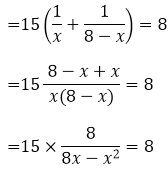⇒ 120 = 8(8x – x2)

⇒ 120 = 64x – 8x2

⇒ 8x– 64x + 120 = 0

⇒ 8(x– 8x + 15) = 0

⇒ x– 8x + 15 = 0

Solving for x by factorization method, we have

⇒ x– 5x – 3x + 15 = 0

⇒ x(x – 5) – 3(x – 5) = 0

⇒ (x – 5)(x – 3) = 0

Now, either x – 5 = 0 ⇒ x = 5

Or, x – 3 = 0 ⇒ x = 3

Thus, the two numbers are 5 and 3 respectively.

10. The sum of a number and its positive square root is 6/25. Find the numbers.

Solution:

Let the number be x.

According to the question, we have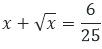Let us assume that x = y2,

So, we get

y2 + y = 6/25

⇒ 25y+ 25y – 6 = 0

⇒ 25y+ 30y – 5y – 6 = 0

⇒ 5y(5y + 6) – 1(5y + 6) = 0

⇒ (5y + 6) (5y – 1) = 0

Now, either 5y + 6 = 0 ⇒ y = -6/5

Or, 5y – 1 = 0 ⇒ y = 1/5

Since, it’s given that only positive square root to be considered we take y = 1/5 only

Thus, x = (1/5)2 = 1/25

Hence, the number is 1/25.

11. The sum of a number and its square is 63/4, Find the numbers.

Solution:

Let the number be x.

So, its square will be x2.

From the question, it’s given that sum of the number and its square is 63/4

Which means,

x + x2 = 63/4

⇒ 4x + 4x2 = 63

⇒ 4x2 + 4x – 63 = 0

Solving for x by factorization method, we have

⇒ 4x2 + 18x – 14x – 63 = 0

⇒ 2x(2x + 9) – 7(2x – 9) = 0

⇒ (2x – 7)(2x + 9) = 0

Now, either 2x -7 = 0 ⇒ x = 7/2

Or, 2x + 9 = 0 ⇒ x = -9/2

Thus, the numbers are 7/2 and -9/2.

12. There are three consecutive integers such that the square of the first increased by the product of the other two gives 154. What are the integers?

Solution:

Let’s consider the three consecutive numbers to be x, x + 1, x + 2 respectively. And x being the first integer of the sequence.

From the question, it’s understood that

x+ (x + 1)(x + 2) = 154

⇒ x+ x+ 3x + 2 = 154

⇒ 2x+ 3x – 152 = 0

Solving for x by factorization method, we have

⇒ 2x+ 19x – 16x – 152 = 0

⇒ x(2x + 19) – 8(2x – 19) = 0

⇒ (2x – 19)(x – 8) = 0

Now, either 2x – 19 = 0 ⇒ x = 19/2 (which is not an integer)

Or, x – 8 = 0 ⇒ x = 8

Hence, considering x = 8 the three consecutive integers are 8, 9 and 10.

13. The product of two successive integral multiples of 5 is 300. Determine the multiples.

Solution:

Given that the product of two successive integral multiples of 5 is 300

Let’s assume the integers be 5x and 5(x+1), where x and x+1 are two consecutive multiples

Then, according to the question, we have

5x[5(x + 1)] = 300

⇒ 25x(x + 1) = 300

⇒ x+ x = 12

⇒ x+ x – 12 = 0

Solving for x by factorization method, we have

⇒ x+ 4x – 3x – 12 = 0

⇒ x(x + 4) – 3(x + 4) = 0

⇒ (x + 4)(x – 3) = 0

Now, either x + 4 = 0 ⇒ x = -4

Or, x – 3 = 0 ⇒ x = 3

For, x = – 4

5x = – 20 and 5(x + 1) = -15

And, for x = 3

5x = 15 and 5(x + 1) = 20

Thus, the two successive integral multiples can be 15, 20 or -15 and -20 respectively.

14. The sum of the squares of two numbers is 233 and one of the numbers is 3 less than twice the other number. Find the numbers.

Solution:

Let one of the number be x. Then the other number will be 2x – 3.

From the question:

x+ (2x – 3)2 = 233

⇒ x+ 4x+ 9 – 12x = 233

⇒ 5x– 12x – 224 = 0

⇒ 5x– 40x + 28x – 224 = 0

⇒ 5x(x – 8) + 28(x – 8) = 0

⇒ (5x + 28) (x – 8) = 0

Now, 5x + 28 cannot be 0

so, x – 8 = 0 ⇒ x = 8

Considering the value of x = 8, we have

2x – 3 = 2(8) – 3 = 16 – 3 = 13

Thus, the two numbers are 8 and 13 respectively.

15. Find the consecutive even integers whose squares have the sum 340.

Solution:

Let’s consider the three consecutive even numbers to be 2x and 2x + 2respectively.

From the question, it’s given that sum of the squares of these integers is 340.

Which means,

(2x)2 + (2x + 2)2 = 340

⇒ 4x2 + 4x2 + 8x + 4 = 340

⇒ 8x2 + 8x – 336 = 0

⇒ 8(x2 + x – 42) = 0

⇒ x2 + x – 42 = 0

Solving for x by factorization method, we have

⇒ x2 + 7x – 6x – 42 = 0

⇒ (x + 7)(x – 6) = 0

Thus, x can be either -7 or 6.

If x = -7, the number are -14 (2x(-7)) and -12 (2x(-7) + 2)

Similarly, if x = 6, the numbers are 12 and 14.

Therefore, the consecutive integers are either -14, -12 or 12, 14.

16. The difference of two number is 4. If the difference of their reciprocals is 4/21, find the numbers.

Solution:

Let the two numbers be x and x – 4 respectively.

Since, given that the difference of two numbers is 4.

Now, from the question, we have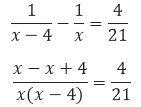⇒ 84 = 4x(x – 4)

⇒ x– 4x – 21 = 0

Solving for x by factorization method, we have

⇒ x– 7x + 3x – 21 = 0

⇒ (x – 7)(x + 3) = 0

Now, either x – 7 = 0 ⇒ x = 7

Or, x + 3 = 0 ⇒ x = -3

Thus, the required numbers are – 3 and 7 respectively.

17. Find two natural numbers which differ by 3 and whose squares have the sum 117.

Solution:

Let the numbers be x and x – 3, as its given the number differ by 3.

From the question, it’s given that sum of squares of these numbers is 117.

x+ (x – 3)= 117

⇒ x+ x+ 9 – 6x – 117 = 0

⇒ 2x– 6x – 108 = 0

⇒ x– 3x – 54 = 0

Solving for x by factorization method, we have

⇒ x– 9x + 6x – 54 = 0

⇒ x(x – 9) + 6(x – 9) = 0

⇒ (x – 9)(x + 6) = 0

Now, either x – 9 = 0 ⇒ x = 9

Or, x + 6 = 0 ⇒ x = – 6

Considering only the positive value of x as natural numbers are always positive i.e, x = 9.

So, x – 3 = 6.

Thus, the two numbers are 6 and 9 respectively.

18. The sum of the squares of three consecutive natural numbers is 149. Find the numbers.

Solution:

Let the three consecutive natural numbers be x, x + 1, and x + 2 respectively.

From the question, we have

x+ (x +1 )+ (x + 2)2 = 149

⇒ x+ x2 + x+ 1 + 2x + 4 + 4x = 149

⇒ 3x2 + 6x – 144 = 0

⇒ x+ 2x – 48 = 0 [dividing by 3 both sides]

Solving for x by factorization method, we have

x2  + 8x – 6x – 48 = 0

⇒ x(x + 8) – 6(x + 8) = 0

⇒ (x + 8)(x – 6) = 0

Now, either x + 8 = 0 ⇒ x = – 8

Or, x – 6 = 0 ⇒ x = 6

Considering only the positive value of x that is 6 and discarding the negative value as the numbers considered are natural numbers.

So, x = 6, x + 1 = 7 and x + 2 = 8.

Thus, the three consecutive numbers are 6, 7, and 8 respectively.

19. The sum of two numbers is 16. The sum of their reciprocals is 1/3. Find the numbers.

Solution:

Let’s consider one of the two natural numbers as x then the other number will be 16 – x, as its given their sum is 16.

Now, form the question we can form the below equation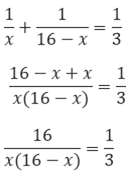⇒ 16x – x2 = 48

⇒ -16x + x+ 48 = 0

⇒ x– 16x + 48 = 0

Solving for x by factorization method, we have

⇒ x– 12x – 4x + 48 = 0

⇒ x(x – 12) – 4(x – 12) = 0

⇒ (x – 12)(x – 4) = 0

So, either x – 12 = 0 ⇒ x = 12

Or, x – 4 = 0 ⇒ x = 4

Thus, the two numbers are 4 and 12 respectively.

20. Determine two consecutive multiples of 3 whose product is 270.

Solution:

Let the two consecutive multiples of 3 be 3x and 3x + 3

From the question, it’s given that

3x*(3x + 3) = 270

⇒ x(3x + 3) = 90 [Dividing by 3 both sides]

⇒ 3x+ 3x = 90

⇒ 3x+ 3x – 90 = 0

⇒ x+ x – 30 = 0

Solving for x by factorization method, we have

x+ 6x – 5x – 30 = 0

⇒ x(x + 6) – 5(x + 6) = 0

⇒ (x + 6)(x – 5) = 0

Now, either x + 6 = 0 ⇒ x = – 6

Or, x – 5 = 0 ⇒ x = 5

Considering the positive value of x, we have only

x = 5, so 3x = 15 and 3x + 3 = 18.

Thus, the two consecutive multiples of 3 are 15 and 18 respectively.

21. The sum of a number and its reciprocal is 17/4. Find the number.

Solution:

Let the number be x.

Then from the question, we have

x + 1/x = 17/4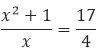⇒ 4(x2+1) = 17x

⇒ 4x+ 4 – 17x = 0

⇒ 4x+ 4 – 16x – x = 0

⇒ 4x(x – 4) – 1(x – 4) = 0

⇒ (4x – 1)(x – 4) = 0

Now, either x – 4 = 0 ⇒ x = 4

Or, 4x – 1 = 0 ⇒ x = 1/4

Thus, the value of x is 4.